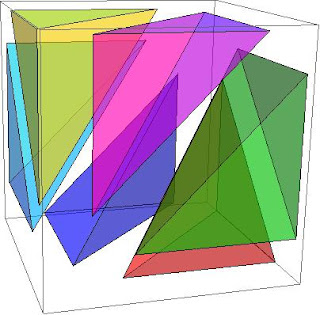## Friday, 15 June 2012

### Visualisation of Kuhn Triangulations

One of the things that got briefly referred to in the under-actuated robotics course was the excellent paper "Variable Resolution Discretization in Optimal Control" by Munos & Moore. I'd had a copy of the paper lying around for a while, because it was mentioned in Steven Lavalle's book Planning Algorithms

That paper recommends a more efficient method of interpolation using simplexes rather than hypercubes. The advantage is that simplex interpolation requires (n+1) points rather than 2^n points in n-dimensions.

One of the best things about the Munos & Moore paper is a really great explanation of how interpolation in simplexes using Kuhn triangulation works, as well as a number of really great diagrams. I've attached some (pretty horrible) Mathematica code that I've used to reproduce something similar to one of the explanatory figures from that paper.

```(* The m-th cartesian aligned basis vector in an n dimensional space. *)
BasisVector[n_, m_] := Map[If[# == m, 1, 0] &, Range[n]]

(* Takes an ordered list of basis functions which describe the order \
to walk the tetrahedra boundary in *)
SimplexVecs[perm_] := Accumulate[
Join[
{ConstantArray[0, Length[perm]]},
Map[BasisVector[Length[perm], #] &, perm]
]
]

(* Generate all 3d tetrahedra on a unit interval *)
TetrahedraGeom3D[] := Map[
GraphicsComplex[
SimplexVecs[#],
Polygon[Subsets[Range[n + 1], {n}]]
] &,
Permutations[Range[n]]
];

SimplexCenter[gc_] := Mean[gc[]]

(* Generate all the geometries *)
gs = TetrahedraGeom3D[];

(* Shift them a little bit away from the cube center*)
gs = Map[
Translate[#, SimplexCenter[#] - {1/2, 1/2, 1/2}] &,
gs
];

(* Generate a 'nice' list of colors *)
mycolors = {Red, Green, Blue, Cyan, Magenta, Yellow};

(* Compose the graphics together, and render *)
Show[MapThread[
Graphics3D[{Opacity[0.4], #2, #1}] &,
{gs, mycolors}
]]
```